Orderless

For the input below (f) has the Orderless attribute which causes (f) to sort
it's arguments into canonical order.In the next cell we see that numeric arguments aren't necessarily sorted in
numeric order.  Instead they are sorted into canonical order.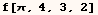Functions with the Orderless attribute sort the arguments before definitions
are applied.  That explains why the result of the input below is not {x, z}
or {z, x}.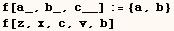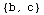The Orderless attribute can effect pattern matching in ways many users don't
understand.  The situation demonstrated in the cells below is one such
example.  The next input clears the definitions and Orderless attribute from
(f).  The rule applied in the next cell can only be used when the first
argument of (f) has the head Real.  For this example the first argument is an
integer so the rule isn't used.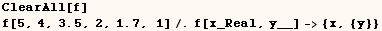For the next input (f) has the Orderless attribute and same rule is applied
again.  This time the arguments are sorted in canonical order before the rule
is used.  Then the pattern matcher finds that the rule matches when
(x→1.7) and (y→ Sequence[1, 2, 3.5, 4, 5]).  The pattern matcher
could have used (x → 3.5) and the appropriate sequence for (y), but the
other match was found first.  Once the pattern matcher found a match it
stopped searching.  Notice the arguments used for (y) in the output are
sorted in canonical order.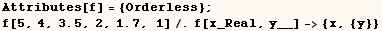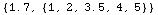Created by Mathematica  (May 16, 2004)

Back to Ted’s Tricks index page